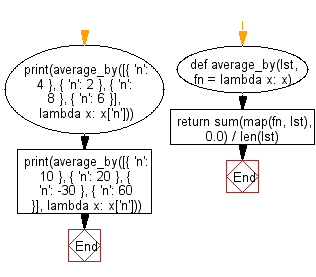﻿ Python: Calculate the average of a given list, after mapping each element to a value using the provided function - w3resource# Python: Calculate the average of a given list, after mapping each element to a value using the provided function

## Python List: Exercise - 238 with Solution

Write a Python program to calculate the average of a given list, after mapping each element to a value using the provided function.

• Use map() to map each element to the value returned by fn.
• Use sum() to sum all of the mapped values, divide by len(lst).
• Omit the last argument, fn, to use the default identity function.

Sample Solution:

Python Code:

``````def average_by(lst, fn = lambda x: x):
return sum(map(fn, lst), 0.0) / len(lst)

print(average_by([{ 'n': 4 }, { 'n': 2 }, { 'n': 8 }, { 'n': 6 }], lambda x: x['n']))
print(average_by([{ 'n': 10 }, { 'n': 20 }, { 'n': -30 }, { 'n': 60 }], lambda x: x['n']))
```
```

Sample Output:

```5.0
15.0
```

Flowchart:## Visualize Python code execution:

The following tool visualize what the computer is doing step-by-step as it executes the said program:

Python Code Editor:

Have another way to solve this solution? Contribute your code (and comments) through Disqus.

What is the difficulty level of this exercise?

Test your Programming skills with w3resource's quiz.

﻿

## Python: Tips of the Day

Clamps num within the inclusive range specified by the boundary values x and y:

Example:

```def tips_clamp_num(num,x,y):
return max(min(num, max(x, y)), min(x, y))
print(tips_clamp_num(2, 4, 6))
print(tips_clamp_num(1, -1, -6))
```

Output:

```4
-1
```